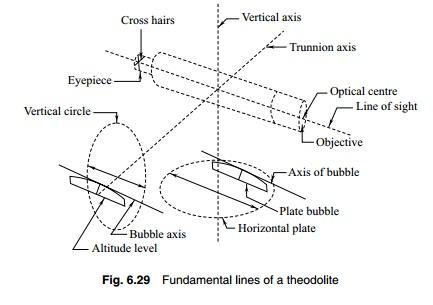Home | | Surveying I | | Surveying | Locating Landscape Details with the Theodolite

Locating Landscape Details with the TheodoliteIn most surveys, it is necessary to locate details such as buildings, railway lines, canals, and other landmarks along with the survey. A transit with a steel tape is used to locate details, and many methods are available, as the transit is an angle-measuring instrument.

Locating Landscape Details with the Theodolite

We have discussed so far methods to survey the main frame or the skeleton of the survey. In most surveys, it is necessary to locate details such as buildings, railway lines, canals, and other landmarks along with the survey. A transit with a steel tape is used to locate details, and many methods are available, as the transit is an angle-measuring instrument. The following methods can be used.

Angle and distance from a single station

A point can be located with an angle to the station along with the distance from that station as shown in Fig. 6.28(a). The angle is preferably measured from the same reference line to avoid confusion. A sketch with the line and the distance and angle measured will help in plotting later. A road can be located as shown in Fig. 6.28(b). Angles to a number of points are measured and with each angle two distances are measured to locate the road.

Angle from one station and distance from another

If for any reason, it is not possible to measure the angle and distance to an object from the same point, it may be possible to locate the point by measuring angles from one station and distances from the other. The recorded data should clearly indicate the stations from which the angle and distance are measured. Figure 6.28(c) shows this method of measuring. The angle is measured from station A to point P. When the instrument is shifted to B, the distance to point P is measured from B with a steel tape.

Angles from two stations

If for some reason, it is not possible to measure distances, then angles from two stations are enough to locate a point. As shown in Fig. 6.28(d), the point P is located by measuring angles to point P from stations A and B.

The following are the fundamental lines.

1.  The vertical axis

2.  The horizontal or trunnion axis

3.  The line of collimation or line of sight

4.  Axis of altitude level

5.  Axis of plate level

The meaning of these terms has been discussed earlier. The axes are shown in Fig. 6.29. When the instrument is properly adjusted, the relationships between these axes are the following.

(a)  The horizontal axis must be perpendicular to the vertical axis.

(b) The axis of the plate level must be perpendicular to the vertical axis.

(c)  The line of collimation must be at right angles to the horizontal axis.

(d) The axis of the altitude level (and telescope level) must be parallel to the line of collimation.

(e)  The vertical circle vernier must read zero when the line of sight is horizon-tal.

Each one of these relations gives conditions for accurate measurement.

(a)  When the horizontal axis is perpendicular to the vertical axis, the line of sight generates a vertical plane when transited.

(b) When the axis of the plate level is perpendicular to the vertical axis, the vertical axis will be truly vertical when the bubble traverses.

(c)  When the line of collimation is at right angles to the horizontal axis, the tele-scope when rotated about the horizontal axis will move in a vertical plane.

(d) When the line of collimation and the axis of altitude level are parallel, the vertical angles will be measured without any index error.

(e)  The index error due to the displacement of the vernier is eliminated when the vernier reads zero with the line of collimation truly horizontal.Study Material, Lecturing Notes, Assignment, Reference, Wiki description explanation, brief detail

Related Topics